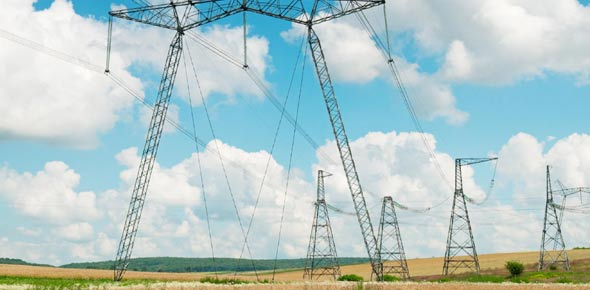13 Questions | Total Attempts: 14SettingsRelated Topics
• 1.
Demand reflects the ____________ amount of work required to perform the function desired.
• A.

Instantaneous

• B.

Necessary

• C.

Regular

• D.

Historical

• 2.
Many vertically-integrated utilities are being forced to separate into distinct G__________, T_________, D_________ and R_________ service companies. (Please enter all four words seperating by comma's)
• 3.
And in order for this electricity to be supplied to the bulb, 60 watts of _______________ must be available at the generator and along the entire path between the bulb and the _______________ _________.
• A.

Capacity, generation source

• B.

Usage, transmission lines

• C.

Demand, supplier

• D.

Capacity, transmission lines

• 4.
1 kilowatt (kW) = ________ watts
• 5.
1 _______ (_W) = 1,000 kW
• 6.
1 gigawatt (GW) = _______ watts
• 7.
1 kilowatt-hour (kWh) = _______ watt-hours
• 8.
1 megawatt hour (kWh) = ________ kWh
• 9.
1 gigawatt hour (gWh) = _________ MWh
• 10.
If you leave the 60-watt buld on for a 24-hour period, it would consume ______ watt-hours. Which is the same as _______ kWh.
• A.

1200,12

• B.

4800, 4.8

• C.

1440, 1.44

• D.

2440, 2.44

• 11.
_____ _______ refers to the greatest amount of electricity required at any given moment.
• 12.
When you turn on a lamp, the bulb creates a _________ of 60 watts.
• A.

Supply

• B.

Capacity

• C.

Demand

• D.

Charge

• 13.
A 60-watt bulb uses ____ watt-hour(s) of energy if you leave it on for one hour.
• A.

1

• B.

6

• C.

600

• D.

60